# Shear force distribution

richard9678
I don't get this shear force distribution stuff.

In my drawing A is sort of a free body diagram. Load W is dead center of a beam, and of course this force is supported at either end by two reaction forces.with a value of W/2.

B is a shear force diagram. For points < L/2, shear force is positive and > L/2 is negative. I believe this is the right diagram. I have a book that shows the reverse, but I think it is incorrect. I don't get this diagram at all, why the sudden change in shear force direction.

C attempts to show how you would show a shearing force. S is shown as half an arrow and pointing upwards. I'm not certain that the arrow points in the correct direction, but if this arrow shows the situation with the shear force diagram, maybe it does. If B is correct.

As I understand it, the reaction force on the left, where the support is, with a value of W/2 is opposed by a force -W/2. acting opposite, to achieve equilibrium. This gives a sense, near the middle on the left hand side, that S is something of a reaction force to some force of -W/2 above it.

I have the equation, W/2 + S = 0. S comes to W/2, but I'm not sure whether S is a reaction force or an action force. But, in my diagram C, that force looks like a reaction force acting against some force of -W/2 above it.

For some reason I just don't grasp,where at a point > L/2, the shear force diagram flips.

The equation given is W/2 + S - W = 0
S = W -(W/2)
S = -W/2. I don't grasp that. Shear force is -W/2.

I would guess, that S is a reaction force, when it's positive, because it's going upwards as it does for a section. But, S turns negative (for some reason) and then S appears like a action force. Does S turn from one to another? What is changing when shear force S flips?.

If a length to a point was greater than L/2, would the free body diagram look like D, E or G? Or what?

I'm perplexed. Thanks.

#### Attachments

•shear force distribution.jpg
15.5 KB · Views: 500
Last edited:

Gold Member
You have a beam with a load and reaction forces in equilibrium. Now imagine you cut that beam somewhere. You now have 2 beams. The question is: what are the forces and moments needed for those beams that need to be added such that each beam is still in equilibrium? Those are the shear force and bending moment. It is like finding the new reaction forces for each beam. No matter where you are going to cut, the shear force acting on one beam will necessarily be the reaction force of the other beam. The following image should help see that: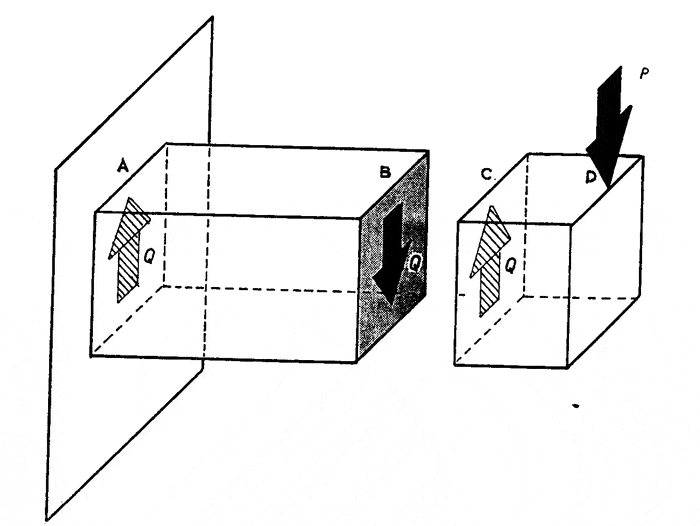The «true» reaction forces are at point A & D. But if you cut the beam anywhere, you end up with 2 ends (B & C) which have an equal and opposite shearing force such that no matter which beam you look at, it will still be in equilibrium. Beam CD pushes down on beam AB and beam AB pushes up on beam CD; It is all relative to your point of view.

This is why there is a negative sign with shear forces. It is not a negative value, it is a change in direction. Whether it is positive or negative, depends on your starting point: Did your start from the left part of the beam or the right part of the beam? There are no wrong answers, you only need to specify where you started.

The shear force diagram answers the following question:

If I start at the left (or right) end, cut the beam at point x (where x vary from 0 to L) and forget about the remaining of the beam, what will be the shear force needed to keep equilibrium?

In the case you presented, it is easy to see that the answer will be the same for any point between 0 and L/2, i.e. -W/2 (if we assume going up is +ve). Once you cross L/2, you now have to include -W in the analysis. The shear force needed for equilibrium from L/2 to L becomes now W/2 for any point on that portion of the beam.

If you would start at the other end (from L to 0), only the sign would change because you are now considering the shear forces from the other beam's point of view.

richard9678
Here is a second drawing. What I can literally see from the drawing is why the direction of shear changes, from positive to negative.

In the first instance of x1 the face of the part is moving in a positive direction. In the second instance x2 the face is on a downwards direction or negative direction. So, just looking the way the faces are moving I can see, I can conclude the direction of shear force. First has positive shear, the second negative shear. The reactions are opposite signs.

What I cannot grasp yet, is the math associated with this matter.

I have seen items 1 and 4 in books. I put item 2 in myself. 3 is just W/2

What exactly are the terms of the equations?

Item 1: V (shear force) when x is less than L/2 = W (Of course shear force isn't W it's W/2)

Item 2: V (shear force) when x is greater than L/2 = -W (Of course shear force isn't -W it's -W/2)

Item 3: W/2 Just half of what W is.

Item 4: I don't get this equation. I know that past the center line, shear is negative to a magnitude of W/2. I don't understand what -W represents. Or, more accurately, why it's included.

So, a positive shear force of a magnitude W/2 acts up the the center line. Balanced by a downward force of -W/2.

A negative shear force acts beyond the center line of magnitude -W/2. Balanced by an upward force of W/2.

"Once you cross L/2, you now have to include -W in the analysis." This is what I'm not getting. My mind goes blank when I try to grasp this. And that is why I just don't grasp item 4. It's something simple I know, but I cannot make out that equation. I think it needs someone to spell it out with words, saying what all the terms mean and the overall thing that is being calculated.

At the moment, my only source for knowing the direction of a shear force is by way of visual of a drawing. It's not by way of math. Items 1 and 2 have some merit, but don't actually give you the magnitude of the shear force. I suppose item 4 starts out "The shear force..."

#### Attachments

Last edited:
richard9678
"Once you cross L/2, you now have to include -W in the analysis."

Why? Why -W and not just W?

I think I needs words of explanation to make me see this. I've got a certain blindness that needs to be overcome through some sort of verbal explanation.

Gold Member
What you are actually doing, is called a statics analysis. It deals with forces and a force - any force - is a vector. A vector has both a magnitude and a direction. If you define a line of action, say a vertical line, then if a force FA has the same magnitude as FB, and one points up and the other points down, then FA = -FB.

In my previous post I said:
In the case you presented, it is easy to see that the answer will be the same for any point between 0 and L/2, i.e. -W/2 (if we assume going up is +ve). Once you cross L/2, you now have to include -W in the analysis.

Since W is pointing down, it means it is the same as an equivalent force pointing up, but with a negative sign. The negative sign implies a change of direction, not a magnitude. The negative signs comes from cos(180°) - which equals -1 - in the dot product between the force (A in the following figure) and the unit vector (B in the following figure) that we use to define basic direction and scale (i.e. pointing up means positive value):

The scalar projection (or scalar component) of a Euclidean vector A in the direction of a Euclidean vector B is given by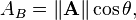where θ is the angle between A and B.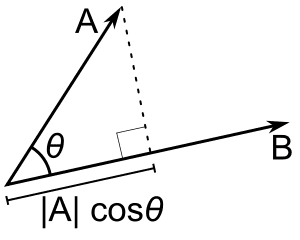source: https://en.wikipedia.org/wiki/Dot_product

Back to your second drawing. Let's just look at the first part of the beam of length x1: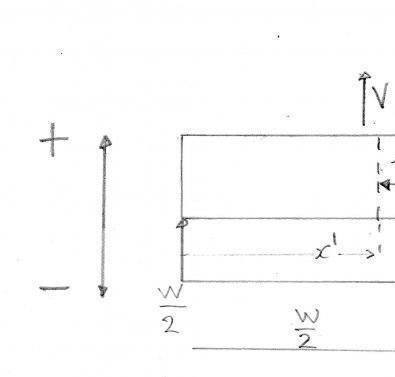We assume +ve is up (per your scale). W/2 is going up, therefore it is +ve. What should be the direction and magnitude of V to balance for W/2 ? The magnitude must be W/2 and for the direction it must point down, hence a negative sign, such that V + W/2 = 0 or V = -W/2.

For the second part of the beam of length x2: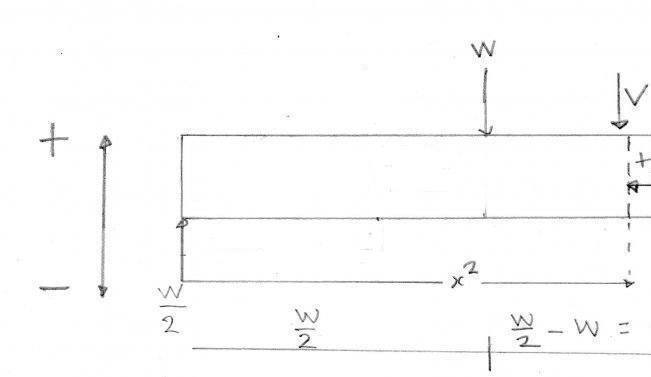We still assume +ve is up (per your scale). W/2 is going up, therefore it is +ve. We now also have W, but it is going down, thus it must be seen as being negative. What should be the direction and magnitude of V to balance those 2 forces? The magnitude must still be W/2 but for the direction it must point up, hence a positive sign, such that V + W/2 + (-W) = 0 or V = W/2.

If you would redo the same exercise and start from the right end, you would get the same magnitudes, but with opposite signs for each section of the beam.